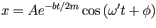# Damped oscillations - Finding damping coefficient

## Homework Statement

A 50.0g hard-boiled egg moves on the end of a spring with force constant k = 25.0 N/m. It is released with an amplitude 0.300m. A damping force Fx = -bv acts on the egg. After it oscillates for 5.00s, the amplitude of the motion has decreased to 0.100m.
Calculate the magnitude of the damping coefficient b.

## Homework Equations## The Attempt at a Solution

I honestly have no idea how to approach this question. Could someone steer me in the right direction?

Anyone?

Redbelly98
Staff Emeritus
Homework Helper

## Homework EquationsNote that the amplitude, at time t, is given by

Amplitude = A e-bt/2m